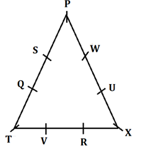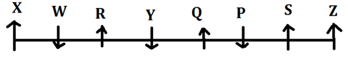Latest Banking jobs   »

# Reasoning Ability Quiz For IBPS RRB PO Clerk Prelims 2022- 18th August

Directions (1-5): Answer the questions based on the information given below.
Nine persons (P, Q, R, S, T, U, V, W, and X) sit around a equilateral triangular table facing towards the center of the table such that three persons sit at the corners and two persons each sit along the three sides of the table but not necessarily in the same order.
P sits 3rd to the left of T who sits at corner. One person sits between P and Q. (From either side of the table). S sits immediate left of Q. U sits 3rd to the right of V. R doesn’t sit at any corners. W sits 2nd to the left of S but not adjacent to T.

Q1. What is the position of V with respect to X?
(a) 3rd to the left
(b) 2nd to the left
(c) 5th to the right
(d) 3rd to the right
(e) None of these

Q2. ___and _____ sits immediate left and 3rd to the right of U respectively.
(a) S, X
(b) X, S
(c) T, X
(d) X, T
(e) None of these

Q3. How many persons sit between R and P when counted from the left of R?
(a) Three
(b) Two
(c) Five
(d) Four
(e) None of these

Q4. Who among the following pair sit adjacent to T?
(a) V, R
(b) R, Q
(c) Q, V
(d) U, X
(e) None of these

Q5. Who sits 5th to the right of R?
(a) P
(b) W
(c) X
(d) T
(e) None of these

Directions (6-10): Answer the questions based on the information given below.
Eight persons (P, Q, R, S, W, X, Y, Z) sit in a linear row such that three persons face towards south direction and remaining persons face towards north direction.
Note: To distinguish between the left and right ends of the row, take the ends as per the north direction but it doesn’t necessarily mean that the persons at the ends face north direction.
Only two persons sit to the left of R. Two persons sit between R and P. P sits 2nd to the left of Z who doesn’t sit adjacent to R. S who sits adjacent to Z, faces same direction as Z. Q who sits 2nd to the left of S faces the same direction as S. Immediate neighbours of Q face same direction. Y sits 3rd to right of X who faces north.

Q6. Who sits 2nd to the left of Y?
(a) P
(b) W
(c) S
(d) R
(e) None of these

Q7. What is the position of Q from extreme right end of the row?
(a) 3rd
(b) 4th
(c) 5th
(d) 6th
(e) None of these

Q8. Who among the following person face towards south direction?
(a) P
(b) Q
(c) R
(d) S
(e) None of these

Q9. How many persons sit between W and P?
(a) One
(b) Two
(c) Three
(d) More than three
(e) None of these

Q10. What is the position of X with respect to R?
(a) 2nd to the left
(b) 2nd to the right
(c) 5th to the right
(d) 4th to the right
(e) Can’t be determined

Directions (11-15): In the following questions assuming the given statement to be true, find which of the conclusion(s) among given conclusions is/are definitely true and then give your answers accordingly.

Q11. Statements: B = C ≤ A = E, E < O < L = T
Conclusions: I. T > A
Il. T = A
(a) Only I is true
(b) Only II is true
(c) Either I or II is true
(d) Neither I nor II is true
(e) Both I and II are true

Q12. Statements: Q > K =T =O, O ≥ E = F ≥ G
Conclusions: I. K > G
Il. K = G
(a) Only I is true
(b) Only II is true
(c) Either I or II is true
(d) Neither I nor II is true
(e) Both I and II are true

Q13. Statements: P > T ≥ U = V ≥ Y, Q> S = P
Conclusions: I. Y < S
II. Q > U
(a) Only I is true
(b) Only II is true
(c) Either I or II is true
(d) Neither I nor II is true
(e) Both I and II are true

Q14. Statements: P ≥ Q < R < S: T > P < W = X
Conclusions: I. X > Q
II. S < W
(a) Only I is true
(b) Only II is true
(c) Either I or II is true
(d) Neither I nor II is true
(e) Both I and II are true

Q15. Statements: A < B ≤ C < D; E < F ≥ D = G
Conclusions: I. G > B
IL. F > A
(a) Only I is true
(b) Only II is true
(c) Either I or II is true
(d) Neither I nor II is true
(e) Both I and II are true

Solutions

Solution (1-5):
Sol.S1. Ans. (b)
S2. Ans. (b)
S3. Ans. (d)
S4. Ans. (c)
S5. Ans. (e)

Solution (6-10):
Sol.S6. Ans. (a)
S7. Ans. (b)
S8. Ans. (a)
S9. Ans. (c)
S10. Ans. (a)

S11. Ans. (a)
Sol. I. T > A – True
Il. T = A – False

S12. Ans. (c)
Sol. I. K > G – False
Il. K = G – False

S13. Ans. (e)
Sol. I. Y < S – True
II. Q > U – True

S14. Ans. (a)
Sol. I. X > Q – True
II. S < W – False

S15. Ans. (e)
Sol. I. G > B – True
IL. F > A – True#### Congratulations!Download Hindu Review of October 2021: Free PDF